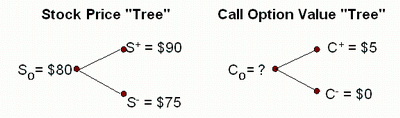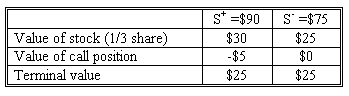### Why should I choose AnalystNotes?

AnalystNotes specializes in helping candidates pass. Period.

##### Subject 1. One-Period Binomial Model
In finance, the binomial options model provides a generalisable numerical method for the valuation of options. It uses a discrete-time framework to trace the evolution of the option's key underlying variable via a binomial lattice (tree), for a given number of time steps between valuation date and option expiration. Each node in the lattice represents a possible price of the underlying, at a particular point in time. This price evolution forms the basis for the option valuation. The valuation process is iterative, starting at each final node and working backwards through the tree to the first node (valuation date), where the calculated result is the value of the option.

Option valuation using this method is a three-step process:
1. Price tree generation.
2. Calculation of option value at each final node.
3. Progressive calculation of option value at each earlier node; the value at the first node is the value of the option.
Definitions:

• r = risk-free rate, assume 1 compounding period
• c+ = Max (0, S+ - X) (call price if the stock price goes up: "up state")
• c- = Max (0, S- - X) (call price if the stock price goes down: "down-state")
• u = (S+ / S0) ("up state" price relative)
• d = (S- / S0) ("down-state" price relative)
• ST = Stock price at time (T)
• π = pi

Formulas:

• π = (1 + r - d) / (u - d) (risk-neutral "up" probability)
• c0 = [π c+ + (1 - π) c-] / (1 + r) (the price of the call option)
• n = (c+ - c-) / (S+ - S-) (the hedge ratio: the number of shares of stock per option to hedge).

We start off by having one binomial period for a European call option.

We assume that the stock price will only take two possible values at the expiration date of the option. In our example:

• Current stock price = S0 = \$80
• Stock price at expiration = \$90 (S+) or \$75 (S-)
• Exercise price of call option = \$85 (X)
• Time to expiration = T = 1/2 year (6 months)
• Risk-free rate of return = r = 6% (discrete and annual)

Based on this information, tree diagrams for the stock value and call option payoffs (state-dependent) would be drawn as follows:The Expectations Approach

In this approach the option value is determined as the present value of the expected future option payouts. The discount rate is the risk-free rate and the expectation is based on the risk-neutral probability measure. In general, European-style options can be valued based on this approach.

Compute risk-neutral probabilities of up and down states.

μ = (90/80) = 1.125
d = (75/80) = 0.9375
π = [(1.06)0.5 - 0.9375] / (1.125 - 0.9375) = 0.4912

Then compute the expected value of the call option.

c0 = [0.4912 \$5 + (1 - 0.4912) \$0] / (1.06)0.5 = \$2.385

Therefore, today's value of the 1-period option is \$2.385.

The No-Arbitrage Approach

This approach is used for option valuation and is built on the key concept of the law of one price, which says that if two investments have the same future cash flows regardless of what happens in the future, then these two investments should have the same current price. Both American-style options and European-style options can be valued based on the no-arbitrage approach.

Step 1. Calculate the hedge ratio (shares per call).

n = (\$5 - \$0) / (\$90 - \$75) = 0.3333

Step 2. Use the hedge ratio to construct a portfolio of stocks and calls in which terminal payoff is state-independent. Let TV denote the terminal value of the portfolio at expiration.

• If stock price = S-, TV- = n S- - c-.
• If stock price = S+, TV+ = n S+ - c+.
• TV- = TV+

Regardless of which way the underlying moves, the portfolio value should be the same (perfectly hedged).

In the example, to form a perfectly hedged portfolio, an investor needs to buy 1/3 of a share of stock for each call that's written (sold), or buy 1 share of stock and sell (write) 3 calls. To see that this is true, consider the position of the portfolio at the expiration of the call if the investor writes 1 call:Step 3. Value the option.

Guaranteed outcome is \$25 for this portfolio, regardless of the value of the stock at expiration. How much should you pay for this risk-free position?

The present value of the guaranteed \$25 to be received in six months is: \$25 / 1.060.5 = \$24.28. To guarantee the \$25 outcome, investor would have to buy 1/3 share of the stock and sell 1 call option.

\$24.28 = n S0 - c0 = initial investment ⇒ c0 = \$80/3 - \$24.28 = \$2.38

This is the same value we ended up with using the expectations approach.

Suppose the option of the previous example is selling for \$3 - a clear case of price not equaling value. Investors would exploit this opportunity by selling the option and buying the underlying. The number of units of the underlying purchased for each option sold would be the hedge ratio: n = (c+ - c-) / (S+ - S-) = 0.3333. Suppose we sell 300 calls and buy 100 shares. The initial outlay would be 100 x \$80 - 300 x \$3 = \$7100. Six months later, the portfolio value will be:

• 100 x \$75 - 0 = \$7500, if stock price = \$75.
• 100 x \$90 - 300 x \$5 = \$7500, if stock price = \$90. Note the 300 here is the number of options bought, not the hedge ratio.

Our six-month return is 7500/7100 - 1 = 5.63%, and the annualized return is (1.0563)2 - 1 = 11.58%. This risk-free return is much higher than the actual risk-free return of 6%.

If the option sells for less than \$2.38, an investor would buy the option and sell short the underlying, which would generate cash up front. At expiration, the investor would have to pay back an amount less than 7%. All investors would perform this transaction, generating a demand for the option that would push its price back to \$2.38.

Therefore, when the option is trading at the price given by the model, a hedge portfolio would earn the risk-free rate.

Single-Period Binomial Put Value

If the option is a put, please note the following differences:

• Hedge ratio n = (p- - p+) / (S+ - S-).
• A risk-free hedge has the same positions in the two instruments (underlying and the put).

User Comment
merc5559 Derivs is brutal
somehow @merc5559: wait until level 2.
Inaganti6 i wonder if i can get to level 2 !
merc5559 On Derivs level 2 now and somehow can see my comment from level 1 on this LOS. Somehow is right. Level 2 Derivatives is even worse.
thebkr777 ^Haha Yeah these are fun... Same section as level 1?
MariaDunet Formula of risk neutral probability comes from assumption that there is no arbitrage and in any case price will be U*p+D*(1-p)=1+r =>
(U-D)*p+D=1+r =>
p=(1+r-D)/(U-D)
The further D or U from 1+r, the less probability would be, eg for 1+r=15 probability of 10 is less than 13.
Allen88 Formula of risk neutral probability comes from assumption that there is no arbitrage and in any case price will be U*p+D*(1-p)=1+r =>
(U-D)*p+D=1+r =>
p=(1+r-D)/(U-D)
The further D or U from 1+r, the less probability would be, eg for 1+r=15 probability of 10 is less than 13.
jperez049 Thanks Allen88
bushi Valuing a derivative via risk-free hedging is equivalent to computing the discounted expected payoff of the option using risk-neutral probabilities rather than actual probabilities.